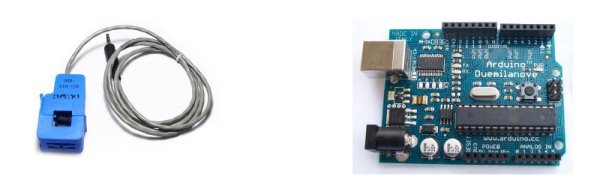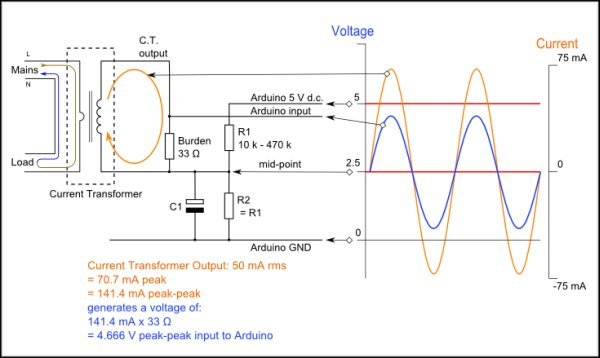# CT sensors – Interfacing with an Arduino

To connect up a CT sensor to an Arduino, the output signal from the CT sensor needs to be conditioned so that it meets the input requirements of the Arduino analog inputs: a positive voltage between 0V and the ADC reference voltage.Note: This page give the example of an Arduino board working at 5 V and of the EmonTx working at 3.3 V. Make sure you use the right supply voltage and bias voltage in your calculations that correspond to your setup.

This can be achieved with the following circuit which consists of two main parts:

1. The CT sensor and burden resistor
2. The biasing voltage divider (R1 & R2)

### Calculating a suitable burden resistor size

If the CT sensor is a current output type such as the YHDC SCT-013-000, the current signal needs to be converted to a voltage signal with a burden resistor. If it is a voltage output CT you can skip this step and miss out the burden resistor as the burden resistor is already built in to the CT.

1) Choose the current range you want to measure

The YHDC SCT-013-000 CT has a current range of 0 to 100 A so for this example let’s choose 100 A as our maximum current.

2) Convert maximum RMS current to peak-current by multiplying by √2.

`Primary peak-current = RMS current × √2 = 100 A × 1.414 = 141.4A`

3) Divide the peak-current by the number of turns in the CT to give the peak-current in the secondary coil.

The YHDC SCT-013-000 CT has 2000 turns and so the secondary peak current will be:

`Secondary peak-current = Primary peak-current / no. of turns = 141.4 A / 2000 = 0.0707A`

4) To maximise measurement resolution the voltage over the burden resistor at peak-current should be the Arduino analog reference voltage (AREF) divided by 2

If you’re using an Arduino running at 5V: AREF / 2 will be 5 V / 2 = 2.5 V and so the ideal burden resistance will be

`Ideal burden resistance = (AREF/2) / Secondary peak-current = 2.5 V / 0.0707 A = 35.4 Ω`35 Ω is not a common resistor value we have a choice of 39 Ω or 33 Ω. Always choose the next smaller value, or the maximum load current will create a voltage higher than AREF. We recommend going for 33 Ω ±1%. In some cases using 2 resistors in series will be closer to the ideal burden value. The further from ideal the value is, the lower the accuracy will be. In this case, the peak current will results in an analog value of 4.7V (3822 after Analog to digital conversion using a 12bit ADC).

Here are the same calculations as above in a more compact form:

For more detail: CT sensors – Interfacing with an Arduino

#### This Post / Project can also be found using search terms:

• ct current arduino in proteus
• using ct with arduino